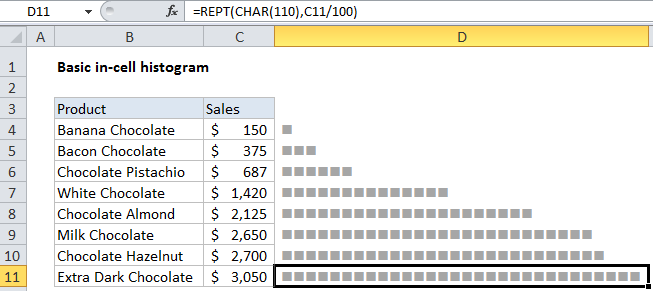## Excel Office

Excel How Tos, Tutorials, Tips & Tricks, Shortcuts

# How to create simple in-cell histogram in Excel

To create a simple in-cell histogram, you can use a formula based on the REPT function. This can be handy when you have straightforward data, and want to avoid the complexity of a separate chart.

## Formula

`=REPT(barchar,value/100)`## Explanation

In the example shown, the formula is:

=REPT(CHAR(110),C11/100)

Worked Example:   Highlight duplicate rows in Excel

### How this formula works

The REPT function simply repeats values. For example, this formula outputs 10 asterisks:

`=REPT("*",10) // outputs **********`

You can use REPT to repeat any character(s) you like. In this example, we use the CHAR function to output a character with a code of 110. This character, when formatted with the Wingdings font, will output a solid square.

`CHAR(110) // square in Wingdings`

To calculate the “number of times” for REPT, we scale values in column C by dividing each value by 100.

`C11/100 // scale values down`

This has the effect of outputting one full square per 100 dollars of sales. Increase or decrease the divisor to suit the data and available space.

Worked Example:   How To Compare Two Lists in Excel

### Alternatively, Conditional formatting can be used

You can also use the “data bars” feature in conditional formatting to display an in cell bar.

Worked Example:   Highlight unprotected cells in Excel